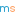# Prize crossnumber, Issue 09

Win £100 of Maths Gear goodies by solving our famously fiendish crossnumberOur original prize crossnumber is featured on pages 54 and 55 of Issue 09.

### Rules

• Although many of the clues have multiple answers, there is only one solution to the completed crossnumber. As usual, no numbers begin with 0. Use of Python, OEIS, Wikipedia, etc. is advised for some of the clues.
• One randomly selected correct answer will win a £100 Maths Gear goody bag, including non-transitive dice, a Festival of the Spoken Nerd DVD, and much, much more. Three randomly selected runners up will win a Chalkdust T-shirt. Maths Gear is a website that sells nerdy things worldwide, with free UK shipping.
• To enter, enter the sum of the across clues below by 9 September 2019. Only one entry per person will be accepted. Winners will be notified by email and announced on our blog by 28 September 2019.

### Crossnumber

Crossnumber #9, set by Humbug:

### Clues

#### Across

• 1. All the digits of this number are equal. (15)
• 8. A factor of 9D. (2)
• 10. A palindrome. (3)
• 12. A prime number. (2)
• 14. The product of the digits of this number is 70. (4)
• 16. All the digits of this number are equal. (15)
• 18. This number is equal to the sum of the fourth powers of its digits. (4)
• 19. Equal to another eight-digit number that appears in this crossnumber. (8)
• 25. An anagram of a palindrome. (5)
• 27. This number is equal to the sum of the cubes of its digits. (3)
• 28. This number is equal to the sum of the cubes of its digits. (3)
• 30. Twice the fourth power of 12A. (5)
• 32. A multiple of 3. (8)
• 35. This number is equal to the sum of the fourth powers of its digits. (4)
• 38. All the digits of this number are equal. (15)
• 42. This number is equal to the sum of the fourth powers of its digits. (4)
• 43. Not equal to 41D. (2)
• 44. This number is equal to the sum of the cubes of its digits. (3)
• 45. A power of two that is one more than a multiple of 9. (2)
• 46. All the digits of this number are equal. (15)

#### Down

• 1. A multiple of 12A. (6)
• 2. An anagram of 3D. (7)
• 3. A multiple of 3 whose second digit is 4. (7)
• 4. An anagram of a palindrome. (6)
• 5. A factor of 10A. (2)
• 6. A multiple of 3. (9)
• 7. Each digit of this number is one more than the previous digit. (8)
• 9. Equal to 10A. (3)
• 11. A multiple of 8A. (3)
• 13. The sum of the digits of 1D.
• 15. Each digit of this number is either one more than or one third of the previous digit. (6)
• 17. A multiple of 5. (3)
• 20. A multiple of 2D. (9)
• 21. Not a multiple of 3. (6)
• 22. A square number. (2)
• 23. The square root of 1 less than 30A. (3)
• 24. Equal to 23D. (3)
• 26. A five-digit number that appears in this crossnumber followed by a three-digit number that appears in this crossnumber. (8)
• 29. Less than 43A. (2)
• 31. A prime number that is equal to the average of the previous and next prime. (3)
• 33. Each digit of this number is either one more or three more than the previous digit. (7)
• 34. Each digit of this number is either one less than or three times the previous digit. (7)
• 36. Not an anagram of a palindrome. (6)
• 37. A three-digit number that appears in this crossnumber followed by a different three-digit number that appears in this crossnumber. (6)
• 39. This number is equal to the sum of the cubes of its digits. (3)
• 40. An anagram of a palindrome. (3)
• 41. A factor of 43A. (2)
• 45. The sum of the digits of 6D. (2)Matthew Scroggs is a PhD student at UCL working on finite and boundary element methods. His website, mscroggs.co.uk, is full of maths and now features a video of him completing a level of Pac-Man optimally.
@mscroggsmscroggs.co.uk    + More articles by Matthew